Smartick is an online platform for children to master math in only 15 minutes a day

Jun23

# Word Problems with Fractions

Today we are going to look at some examples of word problems with fractions.Although they may seem more difficult, in reality, word problems involving fractions are just as easy as those involving whole numbers. The only thing we have to do is:

3. Think about the information we need.
4. Solve it.
5. Simplify, if necessary.
6. Think about whether our solution makes sense (in order to check it).

As you can see, the only difference in fraction word problems is step 5 (simplify).

There are some word problems which, depending on the information provided, we should express as a fraction.  For example:In my fruit basket, there are 13 pieces of fruit, 5 of which are apples.

How can we express the number of apples as a fraction?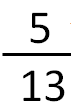5 – The number of apples (5) corresponds to the numerator (the number which expresses the number of parts that we wish to represent).

13 – The total number of fruits (13) corresponds to the denominator (the number which expresses the number of total possible parts).

The solution to this problem is an irreducible fraction (a fraction which cannot be simplified). Therefore, there is nothing left to do.

### Word problems with fractions: involving two fractions

In these problems, we should remember how to carry out operations with fractions.

Carefully read the following problem and the steps we have taken to solve it:Maria spent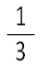of the money her grandparents gave her on an adventure book.  She also spentof the money on a bag of candy.

What fraction of the payment has Maria spent?

We find the common denominator: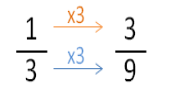We calculate: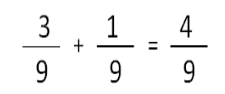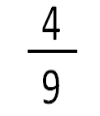### Word problems with fractions: involving a fraction and a whole number

Finally, we are going to look at an example of a word problem with a fraction and a whole number. Now we will have to convert all the information into a fraction with the same denominator (as we did in the example above) in order to calculate

This morning Miguel bought 1 pound of anchovies. In order to eat with his family, he used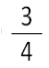of a pound. How much does he have left in the refrigerator?

We convert 1 into a fraction with the same denominator: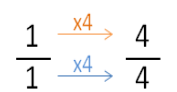We calculate: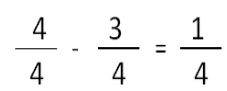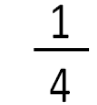What do you think of this post? Do you see how easy it is to solve word problems with fractions?

To keep learning, try Smartick’s free trial.

Latest posts by Smartick (see all)

• Rosalina PerezJun 18 2020, 9:32 PM

A big help for my kids lesson

• PrinceJun 04 2020, 3:39 AM

Thank you for all the homework you have given us. God bless you

• Mie Serira LimoconJan 26 2020, 5:47 AM

Thank you for this problems that involved fractions

• MdickieDec 03 2019, 5:41 PM

Hey I will use this in my game☺

• princess heartJul 29 2019, 7:12 AM

wow nice

• Lea Mae VocalJul 27 2019, 5:14 PM

I like it… but you can level up please 🙄

• Akito swuApr 26 2019, 12:30 PM

Roll two dices, the first dice is the numerator, the second is the denominator, this is the first fraction. Roll both dices again and repeat the process to generate the second fraction. Write a division story problem that incorporates these two fractions.

• Myrtle CraneDec 03 2018, 3:21 AM

Seems easy of the examples but when I have fraction word promblems in front of me then its still hard for me to figure it out.The examples on this site still is helpful.I will use the site that you give on here to get further practice.Thank you for the examples on here

• OliviaJun 06 2019, 10:32 AM

Hello.

• Yetunde GrantNov 23 2018, 2:23 AM

Interesting and very helpful. I’m going to continue using this site and tell others about it too.

• Matcha123Nov 09 2018, 6:49 AM

I really like it

• Ralph (student)Oct 22 2018, 6:50 AM

Hey I am in grade five and it is super helpful for my exams thanks and maybe if you could make more it would be appriciated thx 🙂

• wendyAug 13 2018, 12:22 AM

i kinda like it pls write some more problems